# Dense subset

(Redirected from Dense set)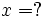This article/section deals with mathematical concepts appropriate for a student in early high school.

If Q is a subset of R (think of R as the real numbers), we say that Q is dense in R if, wherever you are in R, there is a point in Q "infinitely close" to you. Put another way, you can never get any finite distance away from points in Q.

A classic example of a set that is not dense is the integers. If you are at 1.414, there is no integer "infinitely close" to you— the nearest one is .414 away from you.

A classic example (in fact, the classic example) of a set that is dense is the set of rational numbers, that is, fractions of integers. There is a rational that exactly matches 1.414—. But how about a number that is not itself rational, like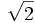? This is where the subtle aspects of "infinitely close" come into play. What this means is that, for any closeness that you want (but not exact equality), there is a rational number that close. If you want a rational number within one thousandth,will do. If you want a rational number within one trillionth,will do.

## In multiple dimensions

Subsets can be dense in two (or more) dimensions. In the plane (the set of points given by two real numbers—the x-coordinate and the y-coordinate), the points with both coordinates rational are dense. If you are at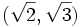, the rational pointis within one-thousandth of where you are.

## More precise definitionThis article/section deals with mathematical concepts appropriate for late high school or early college.

The precise definition of what we meant above by "as close as you want", uses the "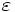" concept used in limits, which is a concept of calculus. Q is dense in R if, for any point x in R and any, there is a point in Q that is withinof x.

## Metric spaces

In more advanced calculus treatments (this subject is often called analysis), denseness can be defined in any metric space. The notion of a point being "withinof x" simply uses the metric.

## Countability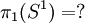This article/section deals with mathematical concepts appropriate for a student in late university or graduate level.

The fact that the rationals are both countable and dense is very important in mathematical analysis. There exist sets (not the reals, of course) that have no countable dense sets.

## Topology

In the language of topology, many of the above concepts are recast in different terms. Q is dense in R if the closure of Q is R.